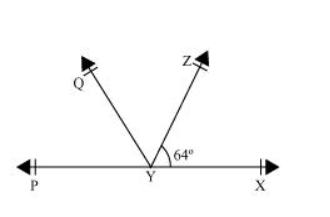# It is given that $\angle X Y Z=64^{\circ}$ and $X Y$ is produced to point $P$. Draw a figure from the given information. If ray $Y Q$ bisects $\angle Z Y P$, find $\angle X Y Q$ and reflex $\angle Q Y P$.

Solution:It is given that line $Y Q$ bisects $\angle P Y Z$.

Hence, $\angle Q Y P=\angle Z Y Q$

It can be observed that $P X$ is a line. Rays $Y Q$ and $Y Z$ stand on it.

$\therefore \angle X Y Z+\angle Z Y Q+\angle Q Y P=180^{\circ}$

$\Rightarrow 64^{\circ}+2 \angle Q Y P=180^{\circ}$

$\Rightarrow 2 \angle Q Y P=180^{\circ}-64^{\circ}=116^{\circ}$

$\Rightarrow \angle Q Y P=58^{\circ}$

Also, $\angle Z Y Q=\angle Q Y P=58^{\circ}$

Reflex $\angle Q Y P=360^{\circ}-58^{\circ}=302^{\circ}$

$\angle X Y Q=\angle X Y Z+\angle Z Y Q$

$=64^{\circ}+58^{\circ}=122^{\circ}$